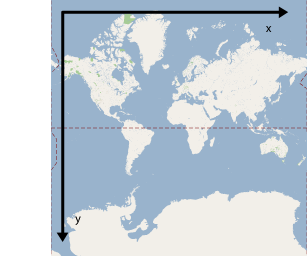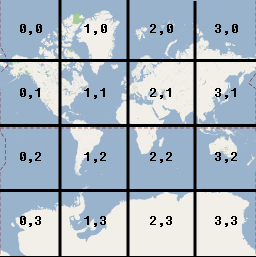It's the 15th anniversary of Google Maps Platform - Check out the latest news and announcements

# Map and Tile Coordinates

The Maps JavaScript API uses the following coordinate systems:

• Latitude and longitude values, which reference a point on the world uniquely. (Google uses the World Geodetic System WGS84 standard.)
• World coordinates, which reference a point on the map uniquely.
• Pixel coordinates, which reference a specific pixel on the map at a specific zoom level.
• Tile coordinates, which reference a specific tile on the map at a specific zoom level.

## World coordinates

Whenever the API needs to translate a location in the world to a location on a map, it first translates latitude and longitude values into a world coordinate. The API uses the Mercator projection to perform this translation.

For convenience in the calculation of pixel coordinates (see below) we assume a map at zoom level 0 is a single tile of the base tile size. We then define world coordinates relative to pixel coordinates at zoom level 0, using the projection to convert latitudes and longitudes to pixel positions on this base tile. This world coordinate is a floating point value measured from the origin of the map projection to the specific location. Note that since this value is a floating point value, it may be much more precise than the current resolution of the map image being shown. A world coordinate is independent of the current zoom level, in other words.

World coordinates in Google Maps are measured from the Mercator projection's origin (the northwest corner of the map at 180 degrees longitude and approximately 85 degrees latitude) and increase in the `x` direction towards the east (right) and increase in the `y` direction towards the south (down). Because the basic Mercator Google Maps tile is 256 x 256 pixels, the usable world coordinate space is `{0-256}, {0-256}`.Note that a Mercator projection has a finite width longitudinally but an infinite height latitudinally. We cut off base map imagery utilizing the Mercator projection at approximately +/- 85 degrees to make the resulting map shape square, which allows easier logic for tile selection. Note that a projection may produce world coordinates outside the base map's usable coordinate space if you plot very near the poles, for example.

You can define your own projection implementing the `google.maps.Projection` interface. (Note that interfaces in the Maps JavaScript API are not classes you subclass but instead are specifications for classes you define yourself.)

## Pixel coordinates

Pixel coordinates reference a specific pixel on the map at a specific zoom level, whereas world coordinates reflect absolute locations on a given projection. Pixel coordinates are calculated using the following formula:

```pixelCoordinate = worldCoordinate * 2zoomLevel
```

From the above equation, note that each increasing zoom level is twice as large in both the `x` and `y` directions. Therefore, each higher zoom level results in a resolution four times higher than the preceding level. For example, at zoom level 1, the map consists of 4 256x256 pixels tiles, resulting in a pixel space from 512x512. At zoom level 19, each `x` and `y` pixel on the map can be referenced using a value between 0 and 256 * 219.

Because we based world coordinates on the map's tile size, a pixel coordinate's integer part has the effect of identifying the exact pixel at that location in the current zoom level. Note that for zoom level 0, the pixel coordinates are equal to the world coordinates.

We now have a way to accurately denote each location on the map, at each zoom level. The Maps JavaScript API constructs a viewport given the zoom level center of the map (as a `LatLng`) and the size of the containing DOM element, and translates this bounding box into pixel coordinates. The API then determines logically all map tiles which lie within the given pixel bounds. Each of these map tiles are referenced using tile coordinates which greatly simplify the displaying of map imagery.

## Tile coordinates

The API cannot load all the map imagery at once for the higher zoom levels. Instead, the API breaks up the imagery at each zoom level into a set of map tiles, which are logically arranged in an order which the application understands. When a map scrolls to a new location, or to a new zoom level, the API determines which tiles are needed using pixel coordinates, and translates those values into a set of tiles to retrieve. These tile coordinates are assigned using a scheme which makes it logically easy to determine which tile contains the imagery for any given point.

Tiles in Google Maps are numbered from the same origin as that for pixels. For Google's implementation of the Mercator projection, the origin tile is always at the northwest corner of the map, with `x` values increasing from west to east and `y` values increasing from north to south. Tiles are indexed using `x,y` coordinates from that origin. For example, at zoom level 2, when the earth is divided up into 16 tiles, each tile can be referenced by a unique `x,y` pair:Note that by dividing the pixel coordinates by the tile size and taking the integer parts of the result, you produce as a by-product the tile coordinate at the current zoom level.

## Example

The following example displays coordinates for Chicago, IL: latitude/longitude values, world coordinates, pixel coordinates, and tile coordinates. Use the zoom control to see the coordinate values at various zoom levels.